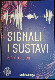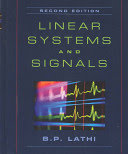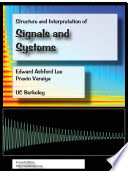### Signals and Systems

#### Course Description

In this course a computational view of signals and systems is implemented and students will acquire basic knowledge necessary in computer engineering, communication, control and electronics. Topics are as follows. Signals as functions. Discrete and continuous time signals. Elementary signal operations. Elementary signals. The four F ourier transforms: CTF S, CTF T, DTF S, DTF T. Fourier transform properties. Sampling and discrete-time signal processing. Discrete time F ourier transform. Systems as functions. Systems with the memory. Linear and time invariant (LTI) systems. Convolution sum and convolution integral. Response of linear time invariant systems. Transfer functions and frequency response. Laplace and z-transform in LTI system analysis. State-space model. Basic structures for the implementation of LTI systems.

#### Learning Outcomes

1. Classify signals
2. Explain and apply tests to unknown systems in order to classify the systems into known categories
3. Interpret and compare the properties of the F ourier Transform, the Laplace Transform and the Z-Transform
4. Apply methods to find response of LTI time discrete and time continuous systems in time domain
5. Apply Laplace and z- transform to find response of LTI time discrete and time continuous systems
6. Analyze transfer functions and frequency responses and present the properties of the systems
7. Analyze MIMO systems described with state equations
8. Analyze and implement linear systems using block diagrams
9. Explain the relation between continuous and discrete signals and systems

#### Forms of Teaching

Lectures

-

Exercises

-

Experiments

-

Consultations

-

Laboratory

-

Continuous Assessment Exam
Type Threshold Percent of Grade Threshold Percent of Grade
Laboratory Exercises 0 % 6 % 0 % 0 %
Homeworks 0 % 10 % 0 % 0 %
Class participation 0 % 4 % 0 % 0 %
Mid Term Exam: Written 50 % 40 % 0 %
Final Exam: Written 50 % 40 %
Exam: Written 51 % 100 %
Exam: Oral 100 %

#### Week by Week Schedule

1. Definition of signals and systems, Classification of signals and systems
2. Signals and systems parameters, Modeling of signals and systems
3. Continuos time Fourier series (CTFS)
4. Continuos time Fourier transform (CTFT)
5. Discrete time Fourier series (DTFS)
6. Discrete time Fourier transform (DTFT)
7. Nyquist frequency, Aliasing in time and frequency domain
8. Interpolation, Signal dimensionality, Midterm exam
9. Impulse response of the LTI systems
10. Convolution sum and integral
11. Linear differential and difference systems
12. Definition, similarities to Laplace transform, Properties
13. Transfer function, Use in solving LTI systems response
15. Parallel, Transformation of state variables, Final exam

#### Study Programmes

[FER3-EN] Electrical Engineering and Information Technology - study
(4. semester)

#### LiteratureBranko Jeren (2021.), Signali i sustavi, Školska knjiga
T. Petković, B. Jeren i ostali (2004.), Signali i sustavi zbirka zadataka, FER, elektroničko izdanjeB. P. Lathi (2004.), Linear Systems and Signals, Oxford University Press
A.V. Oppenheim and A.S. Willsky, with S.H. Nawab (1997.), Signals and Systems, Prentice-HallE.A.Lee, P.Varaiya (2011.), Structure and Interpretation of Signals and Systems, Second Edition, LeeVaraiya.org

#### General

ID 209743
Summer semester
6 ECTS
L2 English Level
L1 e-Learning
60 Lectures
0 Seminar
18 Exercises
15 Laboratory exercises
0 Project laboratory